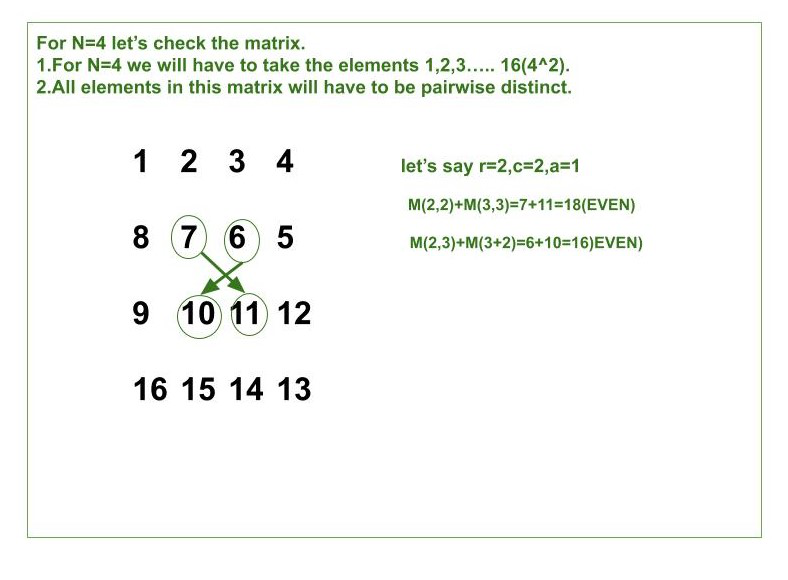# Construct a Matrix whose sum of diagonals for each square submatrix is even

Given an integer N, the task is to construct a matrix M[][] of size N x N with numbers in range [1, N^2], such that the sum of diagonal elements for each square submatrix is even.
Examples:

Input: N = 2
Output:
1 2
4 3
Explanation:
This matrix has 5 square submatrix and 4 of them (, , , ) have a=0 so they satisfy the conditions.
The last square submatrix is the whole matrix M where r=c=a=1. We can see that M(1, 1)+M(2, 2)=1+3=4 and M(1, 2)+M(2, 1)=2+4=6 are both even.
Input: N = 4
Output:
1 2 3 4
8 7 6 5
9 10 11 12
16 15 14 13

Approach: We know that the sum of two numbers is even when their parity is the same. Let us say the parity of M(i, j) is odd that means the parity of M(i+1, j+1), M(i+1, j-1), M(i-1, j+1), M(i-1, j-1) has to be odd.
Below is the illustration for N = 4 to generate a matrix of size 4×4:So from the above illustration we have to fill the matrix in the Checkerboard Pattern. We can fill it in two ways:

• All black cells have an odd integer and white cells have an even integer.
• All black cells have an even integer and white cells have an odd integer.

Below is the implementation of the above approach:

## C++

 `// C++ program for the above approach ` `#include ` `using` `namespace` `std; ` ` `  `// Function to print the desired matrix ` `void` `UniqueMatrix(``int` `N) ` `{ ` `    ``int` `element_value = 1; ` `    ``int` `i = 0; ` ` `  `    ``// element_value will start from 1 ` `    ``// and go up to N ^ 2 ` ` `  `    ``// i is row number and it starts ` `    ``// from 0 and go up to N-1 ` `     `  `    ``// Iterate ove all [0, N] ` `    ``while``(i < N) ` `    ``{ ` `         `  `        ``// If is even ` `        ``if``(i % 2 == 0) ` `        ``{ ` `            ``for``(``int` `f = element_value; ` `                    ``f < element_value + N; f++) ` `            ``{ ` `                 `  `                ``// If row number is even print ` `                ``// the row in forward order ` `                ``cout << f << ``" "``; ` `            ``} ` `            ``element_value += N; ` `        ``} ` `        ``else` `        ``{ ` `            ``for``(``int` `k = element_value + N - 1; ` `                    ``k > element_value - 1; k--) ` `            ``{ ` `                 `  `                ``// If row number is odd print ` `                ``// the row in reversed order ` `                ``cout << k << ``" "``; ` ` `  `            ``} ` `            ``element_value += N; ` `        ``} ` `        ``cout << endl; ` `        ``i = i + 1; ` `    ``} ` `} ` ` `  `// Driver Code ` `int` `main() ` `{ ` `     `  `    ``// Given matrix size ` `    ``int` `N = 4; ` ` `  `    ``// Function call ` `    ``UniqueMatrix(N); ` `} ` ` `  `// This code is contributed by chitranayal `

## Python3

 `# Python3 program for the above approach ` ` `  `# Function to print the desired matrix ` `def` `UniqueMatrix(N): ` ` `  `    ``element_value ``=` `1` `    ``i ``=` `0` ` `  `    ``# element_value will start from 1 ` `    ``# and go up to N ^ 2 ` ` `  `    ``# i is row number and it starts ` `    ``# from 0 and go up to N-1 ` `     `  `    ``# Iterate ove all [0, N] ` `    ``while``(i < N): ` `         `  `        ``# If is even ` `        ``if``(i ``%` `2` `=``=` `0``): ` ` `  `            ``for` `f ``in` `range``(element_value, element_value ``+` `N, ``1``): ` ` `  `                ``# If row number is even print ` `                ``# the row in forward order ` `                ``print``(f, end ``=``' '``) ` `            ``element_value ``+``=` `N ` ` `  `        ``else``: ` ` `  `            ``for` `k ``in` `range``(element_value ``+` `N``-``1``, element_value``-``1``, ``-``1``): ` ` `  `                ``# if row number is odd print ` `                ``# the row in reversed order ` `                ``print``(k, end ``=``' '``) ` ` `  `            ``element_value ``+``=` `N ` ` `  `        ``print``() ` `        ``i ``=` `i ``+` `1` ` `  ` `  `# Driver Code ` ` `  `# Given Matrix Size ` `N ``=` `4` ` `  `# Function Call ` `UniqueMatrix(N) `

Output:

```1 2 3 4
8 7 6 5
9 10 11 12
16 15 14 13
```

Time Complexity: O(N^2)
Auxiliary Space: O(N^2)

Attention reader! Don’t stop learning now. Get hold of all the important DSA concepts with the DSA Self Paced Course at a student-friendly price and become industry ready.

My Personal Notes arrow_drop_upCheck out this Author's contributed articles.

If you like GeeksforGeeks and would like to contribute, you can also write an article using contribute.geeksforgeeks.org or mail your article to contribute@geeksforgeeks.org. See your article appearing on the GeeksforGeeks main page and help other Geeks.

Please Improve this article if you find anything incorrect by clicking on the "Improve Article" button below.

Improved By : chitranayal